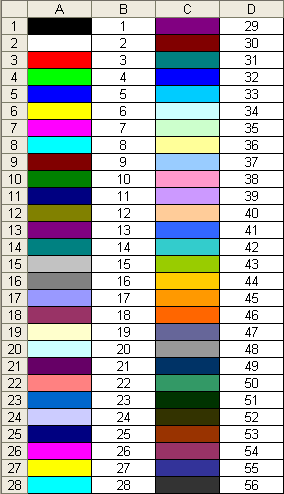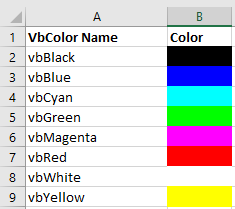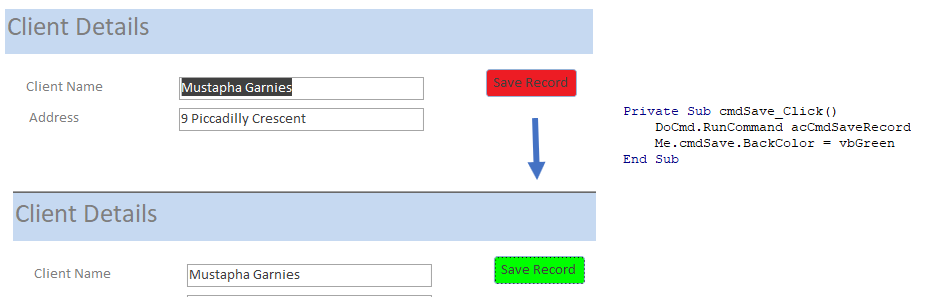# Excel VBA: ColorIndex Codes List & RGB Colors

## VBA Color Index Codes List

When using VBA to code the Colorindex (or background color) of a cell it is useful to know what integer will equal what color. Below is a reference picture which shows the color and lists it’s respective Colorindex. aka VBA Color PaletteHere’s the code to make one for yourself, or just bookmark this page:

``````Sub ColorRef()

Dim x As Integer

For x = 1 To 56
If x < Then
Cells(x, 1).Interior.ColorIndex = x
Cells(x, 2) = x
Else
Cells(x - 28, 3).Interior.ColorIndex = x
Cells(x - 28, 4) = x
End If
Next x

End Sub
``````

### VBA ColorIndex Examples

#### Set Cell Background Color

This example sets the cell’s background color.

``Range("A1").Interior.ColorIndex = 6``

#### Set Cell Font Color

This example sets the cell’s font color.

``Range("A1").Font.ColorIndex = 5``

#### Set Cell Borders Color

This example sets the cell’s border color.

``Range("A1").Borders.ColorIndex = 5``

#### Get Cell Background ColorIndex

This example gets the cell’s background color and assigns it to an Integer variable.

``````Dim col as Integer

col = Range("A1").Interior.ColorIndex``````

#### Set a Cell Background Color to Another Cell’s Color

This example sets a cell color equal to another cell color.

``Range("A1").Interior.ColorIndex  = Range("B1").Interior.ColorIndex``

## VBA Color Property

Instead of using Excel / VBA’s ColorIndex property, you can use the Color property. The Color property takes two input types:

1. vbColor
2. RGB Colors

We will discuss these below:

## VB Color

VB Color is the easiest way to set colors in VBA. However, it’s also the least flexible.  To set a color code using vbColor use the table below:However, as you can see from the table, your options are extremely limited.

#### Set Cell Background Color

``Range("A1").Interior.Color = vbYellow``

#### Set Cell Font Color

``Range("A1").Font.Color = vbBlue``

#### Set Cell Borders Color

``Range("A1").Borders.Color = vbRed``

#### Set a Cell Background Color to Another Cell’s Color

``Range("A1").Interior.Color  = Range("B1").Interior.Color``

## RGB Colors

RGB stands for Red Green Blue. These are the three primary colors that can be combined to produce any other color. When entering colors as RGB, enter a value between 0 and 255 for each color code.

Here’s an example:

``Range("A1").Interior.Color = RGB(255,255,0)``

Above we’ve set Red = 255 (max value), Green = 255 (max value), and Blue = 0 (min value). This sets the cell background color to Yellow.

Instead we can set the cell font color to purple:

``Range("A1").Interior.Color = RGB(128,0,128)``

There are numerous online tools to find the RGB code for your desired color (here’s one).

## ColorIndex Codes List & RGB Colors in Access VBA

Access uses forms to display data.  You can use the ColorIndex Codes to programmatically change the background color and foreground color of objects in your Access forms.

``````Private Sub cmdSave_Click()
'change the background color of the save button when the record is saved.
DoCmd.RunCommand acCmdSaveRecord
cmdSave.BackColor = vbGreen
End Sub``````